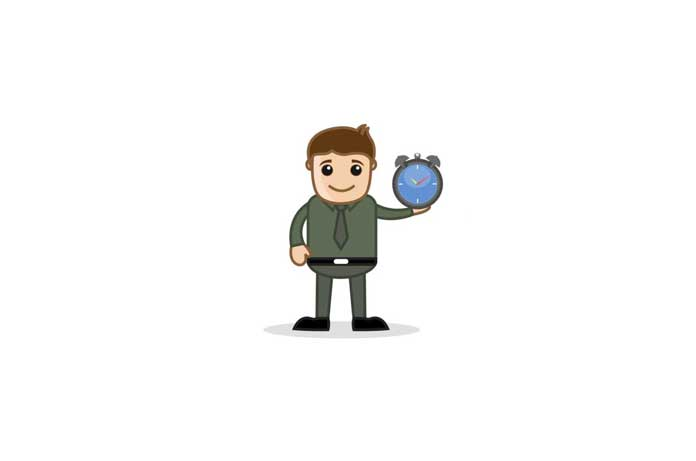Dr. Johnson looked at the clocks on the wall and realised that the number of hours that were left in the day was one-third of the number of hours already passed. How many hours were left in the day?

See the answer

Answer:  6 hours.
Let X be the number of hours left in the day. Then, since there are 24 hours in a day, we have X + 3X = 24. Solving for X, we get X = 6.

Facebook Comments